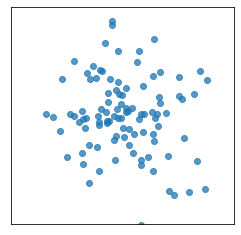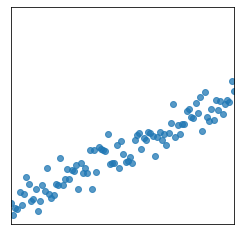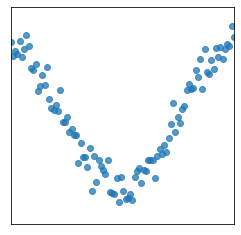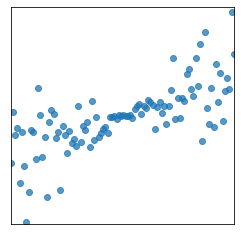# Lecture 12 – Simple Linear Regression¶

by Suraj Rampure

Notebook credits:

• Ani Adhikari
• Data 8's textbook, chapter 15
In :
import numpy as np
import pandas as pd
import matplotlib.pyplot as plt
%matplotlib inline
import seaborn as sns
import plotly.express as px
import plotly.graph_objs as go


### Correlation¶

First, let's come up with some examples of data to use in slides. (Normally this wouldn't be put in the notebook, but it might be of interest to you.)

Also, note here we use np.corrcoef here to compute the correlation coefficients, because we haven't yet defined what r is manually.

In :
# Just noise
np.random.seed(43)
plt.figure(figsize = (4, 4))
plt.xticks([])
plt.yticks([])
plt.xlim(-3, 3)
plt.ylim(-3, 3)
x1, y1 = np.random.randn(2, 100)
plt.scatter(x1, y1, alpha = 0.75);
# plt.savefig('images/s1.png')
print(np.corrcoef(x1, y1))

[[ 1.        -0.1206646]
[-0.1206646  1.       ]]In :
# Strong linear
np.random.seed(43)
plt.figure(figsize = (4, 4))
plt.xticks([])
plt.yticks([])
plt.xlim(-3, 3)
plt.ylim(-3, 3)
x2 = np.linspace(-3, 3, 100)
y2 = x2*0.5 - 1 + np.random.randn(100)*0.3
plt.scatter(x2, y2, alpha = 0.75);
# plt.savefig('images/s2.png')
print(np.corrcoef(x2, y2))

[[1.         0.94882263]
[0.94882263 1.        ]]In :
# Strong non-linear
np.random.seed(43)
plt.figure(figsize = (4, 4))
plt.xticks([])
plt.yticks([])
plt.xlim(-3, 3)
plt.ylim(-3, 3)
x3 = np.linspace(-3, 3, 100)
y3 = 2*np.sin(x3 - 1.5) + np.random.randn(100)*0.3
plt.scatter(x3, y3, alpha = 0.75);
# plt.savefig('images/s3.png')
print(np.corrcoef(x3, y3))

[[1.         0.05201679]
[0.05201679 1.        ]]In :
# Unequal spread
np.random.seed(43)
plt.figure(figsize = (4, 4))
plt.xticks([])
plt.yticks([])
plt.xlim(-3, 3)
plt.ylim(-3, 3)
x4 = np.linspace(-3, 3, 100)
y4 = x4/3 + np.random.randn(100)*(x4)/2.5
plt.scatter(x4, y4, alpha = 0.75);
# plt.savefig('images/s4.png')
print(np.corrcoef(x4, y4))

[[1.         0.70406276]
[0.70406276 1.        ]]## Simple Linear Regression¶

First, let's implement the tools we'll need for regression.

In :
def standard_units(x):
return (x - np.mean(x)) / np.std(x)

def correlation(x, y):
return np.mean(standard_units(x) * standard_units(y))


Let's read in our data.

In :
df = pd.read_csv('galton.csv').iloc[:, 1:]

In :
df

Out:
parent child
0 70.5 61.7
1 68.5 61.7
2 65.5 61.7
3 64.5 61.7
4 64.0 61.7
... ... ...
923 69.5 73.7
924 69.5 73.7
925 69.5 73.7
926 69.5 73.7
927 69.5 73.7

928 rows × 2 columns

An interesting issue is that both our parent and child columns occur at fixed positions. We need to add some random noise, otherwise we'll suffer from gross overplotting.

In :
df['parent'] = df['parent'] + np.random.randn(len(df))/2
df['child'] = df['child'] + np.random.randn(len(df))/2

In :
fig = px.scatter(df, x= 'parent', y = 'child')
fig.show()